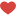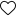As we have seen we have covered a basic understanding of the capabilities of OSMnx in Part I.

Now, let’s take a look at some code examples that demonstrate how to use some of the key features of OSMnx in python.

## Top 5 Features of OSMnx and it’s implementation in Python

### 1. Obtain and visualize drivable, walkable, bikeable, or all street networks from anywhere in the world

If you want to work with street network data for a specific location, OSMnx can be a useful tool.

You first need to import it using the import osmnx as ox command.

`import osmnx as ox`

Once you have imported OSMnx, you can use it to download street network data for a particular location. To do this, you can use the graph_from_place() function and specify the place you want to download data for, as well as the type of network you are interested in (e.g., drivable, walkable, bikeable).

The following code will download the street network data for New York City, NY, USA:

# specify the place you want to download street network data for

`place = 'New York City, NY, USA'`

`G = ox.graph_from_place(place, network_type='drive')`

Now, you can use the plot_graph() function to plot the network as a map, with each node and edge represented by a point and a line, respectively.

# visualize the street network data

`fig, ax = ox.plot_graph(G) `

Using OSMnx, you can easily download and visualize street network data for any location in the world, making it a useful tool for researchers and analysts working with geographic data.

## 2. Visualize a street network as a static map or an interactive leaflet web map

In addition to creating static plots of street networks, OSMnx can also generate interactive web maps using the folium library.

After importing OSMnx and folium and downloading the street network data for a specific location, similar to the previous code:

```import osmnx as ox

import folium```

`G = ox.graph_from_place('New York City, NY, USA')`

You can use the plot_graph_folium() function to generate an interactive web map of the street network using folium. The following code will generate the map:

# generate an interactive leaflet web map of the street network

`m = ox.plot_graph_folium(G)`

Then, you can save the map to an HTML file using the save() method of the folium object. The following code will save the map to an HTML file called “map.html”:

# save the map to an HTML file

`m.save('map.html')`

With just a few lines of code, you can use OSMnx in python and folium to create an interactive web map of a street network, making it a useful tool for exploring and analyzing geographic data.

## 3. Conduct topological and spatial analyses to automatically calculate dozens of indicators

OSMnx can help you conduct topological and spatial analyses and automatically calculate dozens of indicators. These libraries provide a variety of functions for analyzing graphs, including functions for calculating centrality measures, connectivity measures, and spatial statistics.

For example, this code calculates the betweenness centrality of the nodes in a street network.

The betweenness centrality of a node in a network is a measure of how many shortest paths between pairs of other nodes pass through the node. A node with high betweenness centrality is considered to be more central and important in the network, as it acts as a bridge between other nodes.

`import osmnx as ox`

`G = ox.graph_from_place('San Francisco, California, USA')`

You can calculate the betweenness centrality of the nodes in the street network using the following code:

# calculate the betweenness centrality of the nodes

`betweenness = ox.centrality.betweenness_centrality(G)`

This will return a dictionary with the betweenness centrality of each node in the network. You can then loop through the dictionary and print the betweenness centrality of each node, as shown in the following code:

# print the betweenness centrality of each node

```for node, centrality in betweenness.items():

print(f'Node {node}: {centrality:.3f}')```

## 4. Calculate and visualize shortest-path routes

This code downloads street network data for the location of San Francisco. It then uses the shortest_path function from the networkx library to find the shortest path between San Francisco and New York. And creates a figure and axis and displays the figure.

```import osmnx as ox

import networkx as nx

import matplotlib.pyplot as plt```

`G = ox.graph_from_place('San Francisco, California, USA')`

Next, you can define the start and end points for the route using latitude and longitude coordinates.

# define the start and end points for the route

```start = (37.7749, -122.4194)  # latitude, longitude of San Francisco

end = (40.7128, 74.0060)   # latitude, longitude of New York```

The next function takes a network graph object and a list of nodes representing the route as input and plots the route on top of the street network.

# find the shortest path between the start and end points

`route = nx.shortest_path(G, start, end, weight='length')`

The subplots() function creates a figure and a set of subplots (i.e., axes) that can be used to plot data.

# create a figure and axis

`fig, ax = plt.subplots(figsize=(10, 10))`

You can use the following code to plot the street network and the shortest-path route on top of it:

# plot the street network

`ox.plot_graph(G, ax=ax)`

# plot the shortest-path route on top of the street network

`ox.plot_graph_route(G, route, ax=ax)`

# show the figure

`plt.show()`

This will create a figure with the street network plotted in the background, and the shortest-path route plotted on top of it. You can then use the figure to visualize the route and understand the structure of the street network.

This code uses the OSMnx and NumPy libraries to retrieve and analyze bike network data from OpenStreetMap (OSM), including adding node elevations and edge grades to the graph.

```import sys

import numpy as np

import osmnx as ox

import pandas as pd

%matplotlib inline

ox.__version__
```

The following code downloads the street network data for a specific address and constructs a graph object:

```address = "700 Montgomery St, San Francisco, California, USA"

You can add node elevations to the graph object using the following code:

# add node elevations from a single raster file

# some nodes will be null because the single file does not cover the graph’s extents

```raster_path = "./input_data/elevation1.tif"

`G = ox.elevation.add_edge_grades(G, add_absolute=True)`

OSMnx allows you to easily add elevation data to a street network graph object. This can be useful if you are interested in analyzing the elevation profile of a road network, or if you want to use elevation data in further analyses and visualizations.

## Final Thoughts

With its extensive documentation and examples, OSMnx makes it easy for users to get started and conduct their analyses. Whether you are a researcher, planner, or simply interested in exploring and understanding the structure of urban areas, OSMnx is a valuable resource for unlocking the potential of OpenStreetMap data.

Check out more blogs by clicking here.